How to Calculate the Chi-Square Statistic for a Cross Tabulation

In this article, we move on to combine the two to analyze cross tabulations. This article focuses on the chi-square statistic as a way to quantify the relationship between two variables in a cross tabulation.

Key Terms

o         Chi-square statistic

o         Expected frequencies

o         Degrees of freedom

Objectives

o         Understand

o         Use the chi-square statistic to test hypotheses regarding cross tabulations

Resources

o         A more in-depth discussion of cross tabulations and the chi-square statistic is available in a PDF document at http://eclectic.ss.uci.edu/~drwhite/pub/14-2white2.pdf

o         A table of critical values for the chi-square statistic is available at http://www.itl.nist.gov/div898/handbook/eda/section3/eda3674.htm

Let's Begin!

Now that we have gained practice creating and understanding cross tabulations and have reviewed statistical hypothesis testing, we can now analyze cross tabulations using a statistical approach. In this article, we consider several possible methods for determining whether the two variables in a bivariate cross tabulation are related.

Chi-Square Statistic

To avoid making this discussion too vague, we will use an example cross tabulation to illustrate our procedure. As with any such procedure, the reader must be careful to differentiate between the general principles and the specifics of the example. We will use the following cross tabulation as our example; these data reflect the gender and handedness of a number of survey participants.

 Handedness L R Total Gender M 156 472 628 F 185 423 608 Total 341 895 1,236

Although we might be able to guess, simply on the basis of inspection, that these data indicate some relationship between gender and handedness. Nevertheless, we want to find some statistical method of proving that such a conjecture is warranted (or statistically significant). To this end, we introduce the chi-square statistic.

Our first step, following the hypothesis testing procedure, is to formulate a null hypothesis, which we will call H0. For our example, we'll say that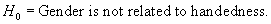The alternative hypothesis is then simply "gender is related to handedness." The second step of the hypothesis testing procedure is to choose a significance level--let's simply select α = 0.05, which is a common value. We are now ready to calculate a test statistic; in this case, we'll use the chi-square statistic. The procedure for calculating this statistic is outlined as follows.

First, we must calculate the expected frequencies, which are the probabilistic number of values we would expect in each data cell, given the values in the total cells. Consider the case of left-handed males: out of 1,236 participants in the survey, 628 were male, and 341 were left handed. The fraction of males, rm, is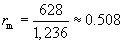Thus, we would expect that this ratio multiplied by the number of left-handed participants (341) should yield the number of left-handed males, or flm.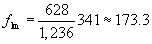Note that the same logic works if we reverse the order of multiplication and first calculate the ratio of left-handed people to the total number of participants and then multiply by the total number of males. In either case, the expected frequency for a given data cell is the product of its corresponding row total and its corresponding column total divided by the grand total. Let's then calculate all the expected frequencies, placing them just below the actual values in each data cell.

 Handedness L Interested in learning more? Why not take an online Applied Statistics course? R Total Gender M 156 173.3 472 454.7 628 F 185 167.7 423 440.3 608 Total 341 895 1,236

Now, we must decide how we can use these expected frequencies to calculate a statistic that helps us determine if a relationship between gender and handedness exists. Such a statistic might involve the differences between the "observed" values (the actual data) and the "expected" values (which we calculated above). But because the sign of the difference is not important, we will square this difference. Furthermore, let's divide each squared difference by its corresponding expected value; this creates something like a proportion rather than a full difference value. Thus, we now create a new table containing these newly calculated values. For left-handed males, we calculate the following: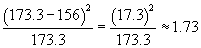Thus,

 Handedness L R Gender M 1.73 0.66 F 1.78 0.68

If we add all of these values, we have something of an aggregate measure of how the observed data values deviate from the expected values; this is the chi-square statistic, which we label χ2.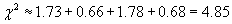We now have a test statistic and its corresponding value for this data set. Our final task is to determine the critical value for this statistic and to determine whether our test statistic value exceeds this critical value. First, recall that we chose 0.05 for our α value. This is a measure of what constitutes a statistically significant deviation. Specifically, α is the probability that the test statistic exceeds the critical value; thus, the smaller the α value that we choose, the less likely the conclusion of our hypothesis test will be incorrect. Using basic probability theory, we can then construct the following equation: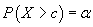This simply states that the probability that our test statistic X exceeds the critical value c is α. Also,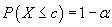This equation is typically what is used to construct tables of values (for the chi-square statistic, for instance). Thus, we use the value 1 – α = 0.95. To find the critical value, the best approach is usually to consult a table of values. Such tables are often available in standard statistics texts as well as online. To use the table, we must also know the number of degrees of freedom of our data (often represented using the variable n). The number of degrees of freedom is actually the number of cell values that must be specified before the remainder are determined by the row and column totals (which we used to calculate expected frequencies, for instance). This number is equal to the product of the number of variable rows minus one and the number of variable columns minus one. In our example, each variable has two possible values, leading to two variable rows and two variable columns. Subtracting one from each and calculating the product, we get unity. This is the number of degrees of freedom.

We can now consult the table to determine the critical value for the example data. We find from the table that c = 3.84. Note that the value of our test statistic, X = χ2 = 4.85, exceeds c. Thus, we might say that with 95% certainty (which is 100% times 1 – α) we can reject the null hypothesis and conclude that according to our data, handedness is related to gender. Note that the null hypothesis was carefully chosen-the assumption was that no relationship between the variables existed. In other words, the expected values were assumed to be close to (or equal to) the observed values, so that if the squared differences became large, our test statistic would exceed the critical value and cause us to reject our initial assumption.

The following practice problem provides the opportunity to practice calculating the chi-square statistic.

Practice Problem: A certain casino game involves numbers between 1 and 32 that each have an associated color (red or black). The cross tabulation for the data is shown below.

 Color Red Black Total Even/Odd Even 7 9 16 Odd 9 7 16 Total 16 16 32

Determine if color has any relation to evenness/oddness.

Solution: We can use hypothesis testing to determine whether such a relationship exists. Let's assume, as our null hypothesis, that color and evenness/oddness are not related, and we'll assume a significance level of α = 0.05. Note that the number of degrees of freedom, n, in this case is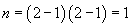Let's calculate the expected frequencies and place them below the observed values in the table. The expected frequency in each case is the product of the corresponding row and column totals divided by the grand total (32).

 Color Red Black Total Even/Odd Even 7 8 9 8 16 , Odd 9 8 7 8 16 Total 16 16 32

Now, let's calculate the values for adding into the chi-square statistic. These component values are the squared differences between the observed and expected values divided by the expected values.

 Color Red Black Even/Odd Even 0.125 0.125 Odd 0.125 0.125

We can now calculate our chi-square statistic.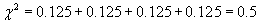From the chi-square table, we find that the critical value for one degree of freedom and 1 – α = 0.95 is 3.84. Thus, since 0.5 < 3.84 (or χ2 < c), we can proceed on the assumption that our null hypothesis is correct--no relationship between color and evenness/oddness exists.

Other Test Statistics for Cross Tabulations

Other test statistics can be calculated for testing hypotheses related to cross tabulations. Although we will not cover any of these statistics here, the same statistical hypothesis testing procedure can be used to evaluate hypotheses using those statistics.